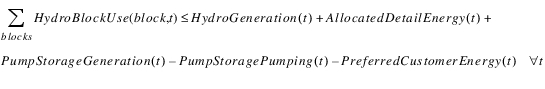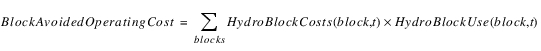Block Economic ValueNoneCalculate Block Economic Value
The economic value of hydropower is set equal to (or replaced by) a piecewise linear function of hydropower generated. The piecewise function can vary by time period. While there is no direct linkage to thermal sources of power, one interpretation of the piecewise linear function is that it represents the coordinated reduction in other power sources as a result of hydropower generation. This method does not attempt to meet an explicit load, rather the load is assumed to be included in the process that generates the piecewise linear functions.
The piecewise linear functions are represented by two slots: maximum of Hydro Block Use slot (same for all t) and Hydro Block Costs. The Hydro Block Costs are the slope of the piecewise linear segments, or alternatively the value of a unit of power. The piecewise linear function is assumed to be concave: the costs are assumed to decrease as the block number increases.
The method name indicates which hydropower resources should be evaluated by the piecewise linear function. Any power sources that precede the “Block” keyword are assumed to already be included in the piecewise linear function and are not evaluated. The order of power sources after the “Block” keyword doesn’t matter for optimization. In this case, the power sources included are as follows:
• Allocated energy
• Conventional hydropower
• Pumped storage units (both pumping and generation)
• Unevaluated: None.
A constraint is added to limit the Hydro Block Use credits to the net generation from all sources minus the Preferred Customer Energy demands that must first be met by the generation.
(5.61)The constraint is written as an inequality rather than an equality to allow for piecewise linearization of power. This constraint is triggered by writing an objective function that includes maximizing Avoided Operating Cost. In addition to this constraint, Avoided Operating Cost is set equal to (or replaced by) the piecewise linear value of power:
(5.62)Slots Specific to This Method
Revised: 08/02/2021